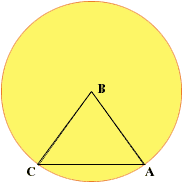My name is Tasha I am a student and this is a secondary question. I have a circle that has an equalateral triangle inscribed in it. The tip of the triangle (B) is at the center of the circle with the other corners (A & C) extending to the sides of the circle. I need to know the equation to find the linear length of AC. I also need to find the cordial length of the circle from point C to A. Hi Tasha, Draw a diagram!Since ABC is an equilateral triangle the length of AC is the same as the length of AB. What is the length of AB? Penny Go to Math Central